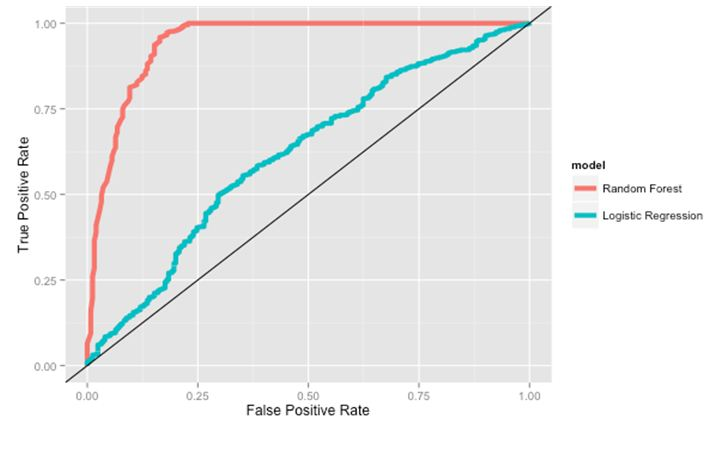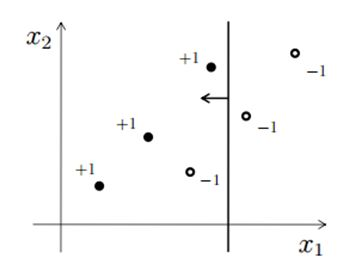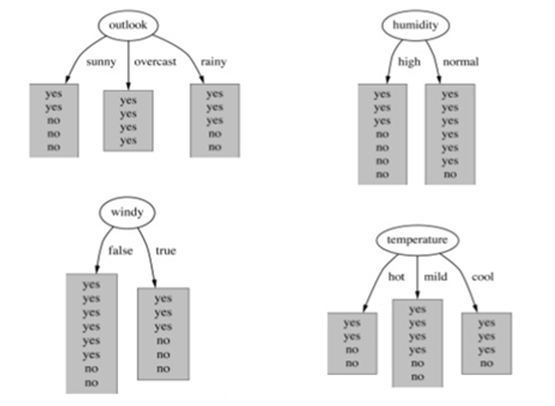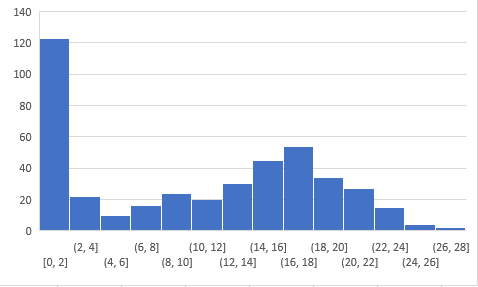# 30 Questions to test a data scientist on Tree Based Models

## Introduction

Decision Trees are one of the most respected algorithm in machine learning and data science. They are transparent, easy to understand, robust in nature and widely applicable. You can actually see what the algorithm is doing and what steps does it perform to get to a solution. This trait is particularly important in business context when it comes to explaining a decision to stakeholders.

This skill test was specially designed for you to test your knowledge on decision tree techniques. More than 750 people registered for the test. If you are one of those who missed out on this skill test, here are the questions and solutions.

Here is the leaderboard for the participants who took the test.

Here are some resources to get in depth knowledge in the subject.

## Skill test Questions and Answers

1) Which of the following is/are true about bagging trees?

1. In bagging trees, individual trees are independent of each other
2. Bagging is the method for improving the performance by aggregating the results of weak learners

A) 1
B) 2
C) 1 and 2
D) None of these

Solution: C

Both options are true. In Bagging, each individual trees are independent of each other because they consider different subset of features and samples.

2) Which of the following is/are true about boosting trees?

1. In boosting trees, individual weak learners are independent of each other
2. It is the method for improving the performance by aggregating the results of weak learners

A) 1
B) 2
C) 1 and 2
D) None of these

Solution: B

In boosting tree individual weak learners are not independent of each other because each tree correct the results of previous tree. Bagging and boosting both can be consider as improving the base learners results.

3) Which of the following is/are true about Random Forest and Gradient Boosting ensemble methods?

1. Both methods can be used for classification task
2. Random Forest is use for classification whereas Gradient Boosting is use for regression task
3. Random Forest is use for regression whereas Gradient Boosting is use for Classification task
4. Both methods can be used for regression task

A) 1
B) 2
C) 3
D) 4
E) 1 and 4

Solution: E

Both algorithms are design for classification as well as regression task.

4) In Random forest you can generate hundreds of trees (say T1, T2 …..Tn) and then aggregate the results of these tree. Which of the following is true about individual(Tk) tree in Random Forest?

1. Individual tree is built on a subset of the features
2. Individual tree is built on all the features
3. Individual tree is built on a subset of observations
4. Individual tree is built on full set of observations

A) 1 and 3
B) 1 and 4
C) 2 and 3
D) 2 and 4

Solution: A

Random forest is based on bagging concept, that consider faction of sample and faction of feature for building the individual trees.

5) Which of the following is true about “max_depth” hyperparameter in Gradient Boosting?

1. Lower is better parameter in case of same validation accuracy
2. Higher is better parameter in case of same validation accuracy
3. Increase the value of max_depth may overfit the data
4. Increase the value of max_depth may underfit the data

A) 1 and 3
B) 1 and 4
C) 2 and 3
D) 2 and 4

Solution: A

Increase the depth from the certain value of depth may overfit the data and for 2 depth values validation accuracies are same we always prefer the small depth in final model building.

6) Which of the following algorithm doesn’t uses learning Rate as of one of its hyperparameter?

2. Extra Trees
4. Random Forest

A) 1 and 3
B) 1 and 4
C) 2 and 3
D) 2 and 4

Solution: D

Random Forest and Extra Trees don’t have learning rate as a hyperparameter.

7) Which of the following algorithm would you take into the consideration in your final model building on the basis of performance?

Suppose you have given the following graph which shows the ROC curve for two different classification algorithms such as Random Forest(Red) and Logistic Regression(Blue)A) Random Forest
B) Logistic Regression
C) Both of the above
D) None of these

Solution: A

Since, Random forest has largest AUC given in the picture so I would prefer Random Forest

8) Which of the following is true about training and testing error in such case?

Suppose you want to apply AdaBoost algorithm on Data D which has T observations. You set half the data for training and half for testing initially. Now you want to increase the number of data points for training T1, T2 … Tn where T1 < T2…. Tn-1 < Tn.

A) The difference between training error and test error increases as number of observations increases
B) The difference between training error and test error decreases as number of observations increases
C) The difference between training error and test error will not change
D) None of These

Solution: B

As we have more and more data, training error increases and testing error de-creases. And they all converge to the true error.

9) In random forest or gradient boosting algorithms, features can be of any type. For example, it can be a continuous feature or a categorical feature. Which of the following option is true when you consider these types of features?

A) Only Random forest algorithm handles real valued attributes by discretizing them
B) Only Gradient boosting algorithm handles real valued attributes by discretizing them
C) Both algorithms can handle real valued attributes by discretizing them
D) None of these

Solution: C

Both can handle real valued features.

10) Which of the following algorithm are not an example of ensemble learning algorithm?

A) Random Forest
C) Extra Trees
E) Decision Trees

Solution: E

Decision trees doesn’t aggregate the results of multiple trees so it is not an ensemble algorithm.

11) Suppose you are using a bagging based algorithm say a RandomForest in model building. Which of the following can be true?

1. Number of tree should be as large as possible
2. You will have interpretability after using RandomForest

A) 1
B) 2
C) 1 and 2
D) None of these

Solution: A

Since Random Forest aggregate the result of different weak learners, If It is possible we would want more number of trees in model building.  Random Forest is a black box model you will lose interpretability after using it.

Context 12-15

Consider the following figure for answering the next few questions. In the figure, X1 and X2 are the two features and the data point is represented by dots (-1 is negative class and +1 is a positive class). And you first split the data based on feature X1(say splitting point is x11) which is shown in the figure using vertical line. Every value less than x11 will be predicted as positive class and greater than x will be predicted as negative class.12) How many data points are misclassified in above image?

A) 1
B) 2
C) 3
D) 4

Solution: A

Only one observation is misclassified, one negative class is showing at the left side of vertical line which will be predicting as a positive class.

13) Which of the following splitting point on feature x1 will classify the data correctly?

A) Greater than x11
B) Less than x11
C) Equal to x11
D) None of above

Solution: D

If you search any point on X1 you won’t find any point that gives 100% accuracy.

14) If you consider only feature X2 for splitting. Can you now perfectly separate the positive class from negative class for any one split on X2?

A) Yes
B) No

Solution: B

It is also not possible.

15) Now consider only one splitting on both (one on X1 and one on X2) feature. You can split both features at any point. Would you be able to classify all data points correctly?

A) TRUE
B) FALSE

Solution: B

You won’t find such case because you can get minimum 1 misclassification.

Context 16-17

Suppose, you are working on a binary classification problem with 3 input features. And you chose to apply a bagging algorithm(X) on this data. You chose max_features = 2 and the n_estimators =3. Now, Think that each estimators have 70% accuracy.

Note: Algorithm X is aggregating the results of individual estimators based on maximum voting

16) What will be the maximum accuracy you can get?

A) 70%
B) 80%
C) 90%
D) 100%

Solution: D

Refer below table for models M1, M2 and M3.

 Actual predictions M1 M2 M3 Output 1 1 0 1 1 1 1 0 1 1 1 1 0 1 1 1 0 1 1 1 1 0 1 1 1 1 0 1 1 1 1 1 1 1 1 1 1 1 0 1 1 1 1 0 1 1 1 1 0 1

17) What will be the minimum accuracy you can get?

A) Always greater than 70%
B) Always greater than and equal to 70%
C) It can be less than 70%
D) None of these

Solution: C

Refer below table for models M1, M2 and M3.

 Actual predictions M1 M2 M3 Output 1 1 0 0 0 1 1 1 1 1 1 1 0 0 0 1 0 1 0 0 1 0 1 1 1 1 0 0 1 0 1 1 1 1 1 1 1 1 1 1 1 1 1 1 1 1 1 1 1 1

18) Suppose you are building random forest model, which split a node on the attribute, that has highest information gain. In the below image, select the attribute which has the highest information gain?A) Outlook
B) Humidity
C) Windy
D) Temperature

Solution: A

Information gain increases with the average purity of subsets. So option A would be the right answer.

19) Which of the following is true about the Gradient Boosting trees?

1. In each stage, introduce a new regression tree to compensate the shortcomings of existing model
2. We can use gradient decent method for minimize the loss function

A) 1
B) 2
C) 1 and 2
D) None of these

Solution: C

Both are true and self explanatory

20) True-False: The bagging is suitable for high variance low bias models?

A) TRUE
B) FALSE

Solution: A

The bagging is suitable for high variance low bias models or you can say for complex models.

21) Which of the following is true when you choose fraction of observations for building the base learners in tree based algorithm?

A) Decrease the fraction of samples to build a base learners will result in decrease in variance
B) Decrease the fraction of samples to build a base learners will result in increase in variance
C) Increase the fraction of samples to build a base learners will result in decrease in variance
D) Increase the fraction of samples to build a base learners will result in Increase in variance

Solution: A

Context 22-23

Suppose, you are building a Gradient Boosting model on data, which has millions of observations and 1000’s of features. Before building the model you want to consider the difference parameter setting for time measurement.

22) Consider the hyperparameter “number of trees” and arrange the options in terms of time taken by each hyperparameter for building the Gradient Boosting model?

Note: remaining hyperparameters are same

1. Number of trees = 100
2. Number of trees = 500
3. Number of trees = 1000

A) 1~2~3
B) 1<2<3

C) 1>2>3
D) None of these

Solution: B

The time taken by building 1000 trees is maximum and time taken by building the 100 trees is minimum which is given in solution B

23) Now, Consider the learning rate hyperparameter and arrange the options in terms of time taken by each hyperparameter for building the Gradient boosting model?

Note: Remaining hyperparameters are same

1. learning rate = 1
2. learning rate = 2
3. learning rate = 3

A) 1~2~3
B) 1<2<3

C) 1>2>3
D) None of these

Solution: A

Since learning rate doesn’t affect time so all learning rates would take equal time.

24) In greadient boosting it is important use learning rate to get optimum output. Which of the following is true abut choosing the learning rate?

A) Learning rate should be as high as possible
B) Learning Rate should be as low as possible
C) Learning Rate should be low but it should not be very low
D) Learning rate should be high but it should not be very high

Solution: C

Learning rate should be low but it should not be very low otherwise algorithm will take so long to finish the training because you need to increase the number trees.

25) [True or False] Cross validation can be used to select the number of iterations in boosting; this procedure may help reduce overfitting.

A) TRUE
B) FALSE

Solution: A

26) When you use the boosting algorithm you always consider the weak learners. Which of the following is the main reason for having weak learners?

1. To prevent overfitting
2. To prevent under fitting

A) 1
B) 2
C) 1 and 2
D) None of these

Solution: A

To prevent overfitting, since the complexity of the overall learner increases at each step. Starting with weak learners implies the final classifier will be less likely to overfit.

27) To apply bagging to regression trees which of the following is/are true in such case?

1. We build the N regression with N bootstrap sample
2. We take the average the of N regression tree
3. Each tree has a high variance with low bias

A) 1 and 2
B) 2 and 3
C) 1 and 3
D) 1,2 and 3

Solution: D

All of the options are correct and self explanatory

28) How to select best hyperparameters in tree based models?

A) Measure performance over training data
B) Measure performance over validation data
C) Both of these
D) None of these

Solution: B

We always consider the validation results to compare with the test result.

29) In which of the following scenario a gain ratio is preferred over Information Gain?

A) When a categorical variable has very large number of category
B) When a categorical variable has very small number of category
C) Number of categories is the not the reason
D) None of these

Solution: A

When high cardinality problems, gain ratio is preferred over Information Gain technique.

30) Suppose you have given the following scenario for training and validation error for Gradient Boosting. Which of the following hyper parameter would you choose in such case?

 Scenario Depth Training Error Validation Error 1 2 100 110 2 4 90 105 3 6 50 100 4 8 45 105 5 10 30 150

A) 1
B) 2
C) 3
D) 4

Solution: B

Scenario 2 and 4 has same validation accuracies but we would select 2 because depth is lower is better hyper parameter.

## Overall Distribution

Below is the distribution of the scores of the participants:

##You can access the scores here. More than 350 people participated in the skill test and the highest score obtained was 28.

## End Notes

I tried my best to make the solutions as comprehensive as possible but if you have any questions / doubts please drop in your comments below. I would love to hear your feedback about the skill test. For more such skill tests, check out our current hackathons.

### Learn, engage,compete, and get hired!

You can also read this article on Analytics Vidhya's Android APP•Carl says:

Hi,

A couple issues with the quiz:

1) #23 refers to changing the learning rate of a Random Forest. Did you mean to ask about a boosting algorithm?

2) Questions #23 through #25 look like the answers are offset by 1 (e.g. “The time taken by building 1000 trees is maximum and time taken by building the 100 trees is minimum which is given in solution B” should be explaining #22 instead of #23).

Thank you.

•Ankit Gupta says:

Yes, You are right this should boosting instead of random forest. And offset is fixed now. Thanks for noticing Carl!

•Pratima Joshi says:

In case of Q 30, does the training error not matter?
Also, the options for answers did not include “5” ! 🙂

•SrikantPanda says:

Hello Ankit,
For qn. 30 can you help me to understand why the answer is not scenario 3 that is of depth 6 with training error 50 validation error 100, as both error seems to be reducing and has less training and validation error.

•Srikant says:

Hello Ankit,
For qn. 30 can you help me to understand why the answer is not scenario 3 that is of depth 6 with training error 50 validation error 100, as both error seems to be reducing and has less training and validation error.

•Ak says:

The video ads on some pages are really annoying. They seem to be newly added. They cause the page to scroll up/down automatically making it impossible to read the content. Please check.

•Data Science Training in Hyderabad says:

Hi Ankit
Good questions and answers are given about the data scientist tree based models.Thank u
Those are really helpful too Data Science users.

•Data Science Training In Hyderabad says:

Hi,
Thanks for sharing such a wonderful article with 30 Questions to test a data scientist on Tree-Based Models
The way your explanation is good
Thank you

•Data Science Training In Hyderabad says:

Hi,
Thanks for sharing such an informative and useful post
We are eagerly waiting for more articles on this blog